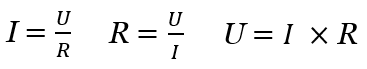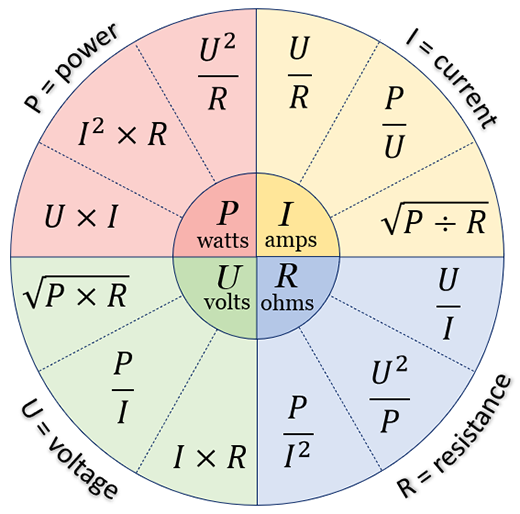# Ohm's Law Calculator

Use this Ohm's Law calculator to easily calculate the power, resistance, voltage, or electrical current, based on two known parameters. Enter any two parameters to calculate the other two. Supports amps, milliamps, watts, kilowatts, megawatts, volts, millivolts and kilovolts, ohms, kiloohms, and megaohms. Formulas, equations, and Ohm's law wheel for reference.

Share calculator:

Embed this tool:
get code

## Using the Ohm's Law Calculator

Using the above multipurpose ohms law calculator, you can:

• Calculate power and resistance given the voltage and current
• Calculate power and current given voltage and resistance
• Calculate power and voltage given current and resistance
• Calculate current and resistance given voltage and power
• Calculate current and voltage given power and resistance
• Calculate voltage and resistance given power and current

Just enter the two known quantities describing the electrical current and the other two will be calculated based on them. The result will be displayed in the metrics chosen with supported metrics being: amperes, milliamperes, milliwatts, watts, kilowatts, megawatts, gigawatts, millivolts, volts, kilovolts, megavolts, ohms, kiloohms, and megaohms. (A, mA, mW, W, KW, MW, GW, mV, V, KV, MV, Ω, KΩ, and MΩ).

Using this tool you can easily calculate ohms to watts, ohms to volts, ohms to amps, volts to ohms, watts to ohms, amps to ohms, and so on.

## Ohm's Law & Formula

Ohm's law states that the current between any two points of an electrical conductor is directly proportional to the voltage across the two points. The relationship is mathematically described by the equations:where I is the current in amperes, V is the voltage in Volts, and R is the resistance in Ohms (Ω). The three are equivalent transformations of the Ohm's law formula and are used in circuit analysis and planning of electrical grids.

Ohm's Law is applicable for electrical circuits containing resistive elements only (capacitors and inductors are not allowed) and it works the same for constant (DC) or time-varying (AC) driving voltage or current.

To calculate electrical power, which is the rate at which electrical energy is transferred through a conductor per unit time, we need to know that P = V x I (from Joule's Law), where P is the power in Watts, V and I according to the above definitions. This basic equation can be transformed into different formulas depending on the known electrical measurements and is how our Ohm calculator works.

## Ohm's Law Wheel

When you combine the formulas you get the Ohm's Law Formula Wheel, shown below, which reflects how our calculator works. The Ohm's Law wheel represents all possible relationships between power (P), resistance (R), current (I) and voltage (V).To use the wheel, select the wanted quantity from the inner circle of the wheel and then the appropriate formula in that quadrant based on what you know about the electrical current you are working with. Even though using a calculator is more convenient, a schematic representation as the above makes it easier to grasp the underlying formulas and equations, and their relationships.